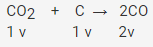# One liter of CO2 is passed through red hot coke

One liter of CO2 is passed through red hot coke. The volume becomes 1.4 liters at same temperature and pressure. The composition of the product is?

The reaction equation is as,Let x lit of CO2 gives 2x lit of CO
Volume left of CO2 = (1-x)
Total volume = 1.4 lit
So,
(1-x) + 2x = 1.4
1+x= 1.4
x= 0.4 lit
As CO = 2x
= 2×0.4
CO = 0.8 lit
Volume of CO2 = 1.4- 0.8
= 0.6 lit
Composition of product is 0.8 lit CO and 0.6 lit of CO2.